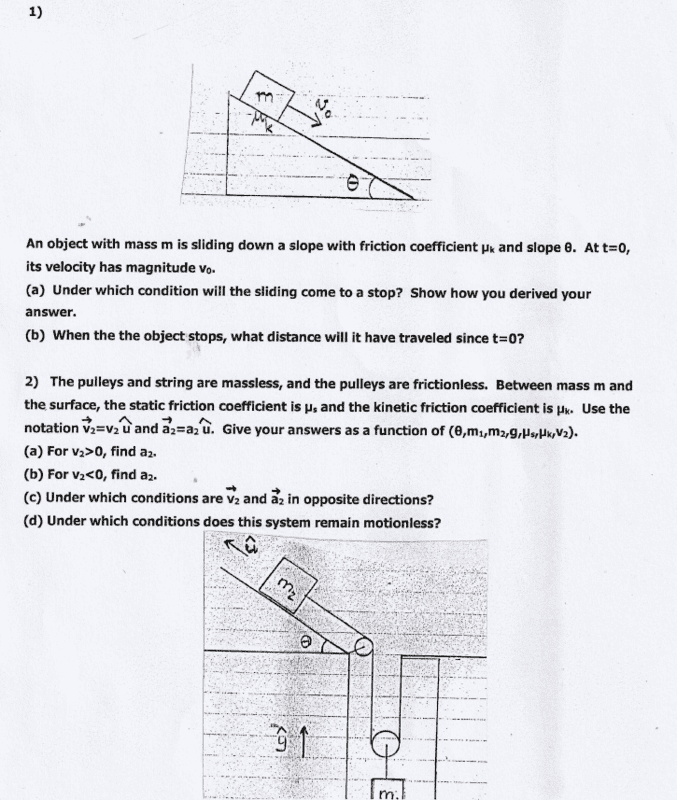# 2 inclined plane problems, one with pulleys

physxishard
Homework Statement:
Relevant Equations:
For the a part of the first problem I did this: mgsinθ < µk*mgcosθ
Sinθ < µk*cosθ
Tanθ < µk so θ should be less than arctan µk
For part b of the first problem I found the net force on x-axis and derived acceleration from there. Then I used that acceleration in Vf^2 = Vi^2 +2ad which gave me the result => d = -V0^2 / 2(gsinθ - µk*gcosθ)
I'm not really sure about these but at least I was able to get results. I'm really having problems with the second question though. I'd really appreciate some help. Thanks in advance.